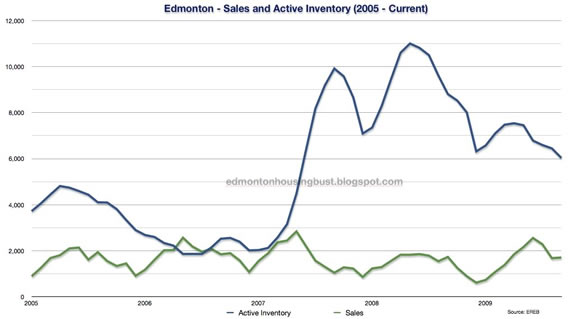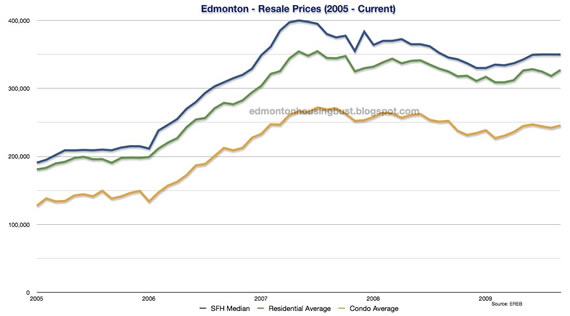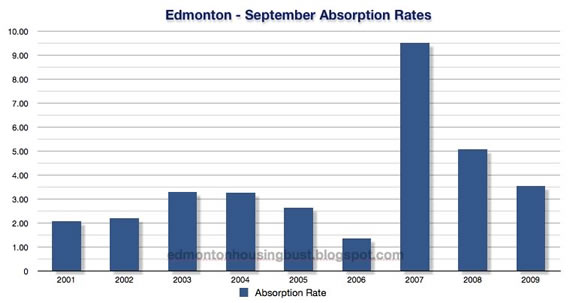# September numbers are in….The September numbers were released today, and after a cooling in August sales held in September. Up slightly from month-over-month and down slightly year-over-year. So, interest rates are continuing to spur spending, which is fine as long as the status quo remains.On the price front, averages were up a couple ticks across the board, while SFH median held. So, we were continuing to see increasing action in the higher price ranges. In the next week or so I’m hoping to do another update on the sales mix for the price ranges.

As I mentioned earlier, we’re also starting to see YoY increases by this time last year prices started dropping, and interest rates this year have proved to be a game changer and created a sense of affordability which has led to a price rebound. Time will tell how sustainable these gains really are.With the seasonal decline in inventory starting to hit and sales holding, absorption rate went down slightly from August to 3.54 from 3.85. Still in the buyers range, but there seems to be a lot of disparity between different sectors. Certain types of property sell very well, but others are very slow.As always, here are the hard goods:

Sales = 1,704
Since two years ago = +635% (+662)
Since one year ago = -1.4% (-25)
Since last month = +1.9% (+31)

Active Listings = 6,032
Since two years ago = -39.2% (-3,886)
Since one year ago = -31.5% (-2,776)
Since last month = -6.4% (-413)

Single Family Homes Median= \$349,900
Since peak (May ’07) = -12.5% (-\$50,100)
Since one year ago = +1.3% (+\$4,400)
Since six months ago = +4.8% (+\$15,900)
Since last month = -0.0% (-\$100)

Residential Average = \$327,235
Since peak (July ’07) = -7.7% (-\$27,483)
Since one year ago = +0.7% (+\$2,330)
Since six months ago = +5.9% (+\$18,203)
Since last month = +2.8% (+\$8,914)

Single Family Homes Average = \$371,947
Since peak (May ’07) = -12.7% (-\$54,081)
Since one year ago = +2.7% (+\$9,850)
Since six months ago = +6.4% (+\$22,231)
Since last month = +1.4% (+\$5,159)

Condo Average = \$245,546
Since peak (July ’07) = -9.7% (-\$26,362)
Since one year ago = -2.7% (-\$6,687)
Since six months ago = +6.5% (+\$15,077)
Since last month = +1.5% (+\$3,511)

Townhome Average = \$299,964
Since peak (Oct ’07) = -18.5% (-\$68,000)
Since one year ago = -5.0% (-\$15,726)
Since six months ago = +8.4% (+\$23,188)
Since last month = +2.0% (+\$5,957)